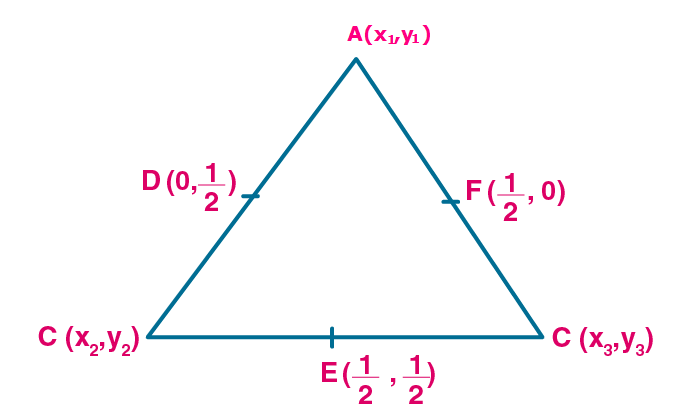Newbie

# Find the coordinates of the vertices of the triangle the middle points of whose sides are (0, 1/2 ) , (1/2 , 1/2) and ( 1/2 , 0).

• 2

M.L Aggarwal book Important Question of class 10 chapter Based on Section Formula for ICSE BOARD.
You have to find the coordinates of the vertices of the triangle the middle points of whose sides are given in this question so solve this question.
This is the Question Number 28, Exercise 11 of M.L Aggarwal.

Share

1.Let A(x1,y1), B(x2,y2) and C(x3,y3) be the vertices of the triangle ABC.

Consider AB

By midpoint formula, (x1+x2)/2 = 0

x1+x2 = 0

x1 = -x2 ..(i)

By midpoint formula, (y1+y2)/2 = ½

y1+y2 = 1 …(ii)

Consider AC

By midpoint formula, (x1+x3)/2 = ½

x1+x3 = 1 …(iii)

By midpoint formula, (y1+y3)/2 = 0

y1+y3 = 0

y1 = -y3 …(iv)

Consider BC

By midpoint formula, (x2+x3)/2 = ½

x2+x3 = 1 …(v)

By midpoint formula, (y2+y3)/2 = ½

y2+y3 = 1 …(vi)

Substitute (i) in (iii)

Then (iii) becomes -x2+x3 = 1

Equation (v) x2+x3 = 1

Adding above two equations, we get

2x= 2

x= 2/2 = 1

Substitute x3 = 1 in (iii), we get x1 = 0

x2 = 0 [From (i)]

So x1 = 0, x2 = 0, x3 = 1

Substitute (iv) in (ii)

Then (ii) becomes -y3+y2 = 1

Equation (vi) y2+y3 = 1

Adding above two equations, we get

2y2 = 2

y2 = 2/2 = 1

Substitute y2 = 1 in (i), we get y1 = 0

y3 = 0

So y1 = 0, y2 = 1, y3 = 0

Hence the Co-ordinates of vertices are A(0,0), B(0,1) and C(1,0).

• 5